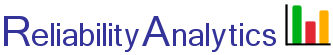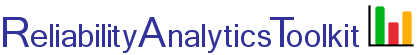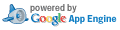# MTBF Test Time Calculator

This tool calculates the test time required to demonstrate a mean time between failure (MTBF) value at a given confidence level. It assumes an exponential failure distribution (i.e., constant unit failure rate) and uses the chi-square distribution, as described in the "Confidence Limits - Exponential Distribution" tool, taking as input the number of allowable failures and required MTBF and solving Equation 2 for T, the required test time. Because the failure rate is constant (i.e., only "chance" failures occur, no wearout), testing one unit for 100 hours is equivalent to testing 10 units for 10 hours each.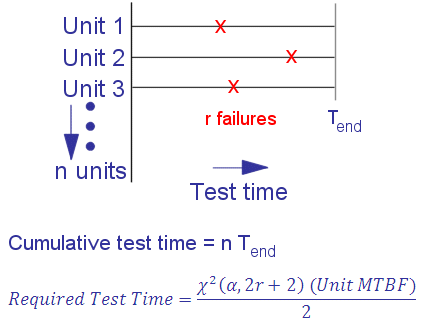## Calculation Inputs:

1. Select method:
Method 1
Method 2
2. Unit MTBF requirement (hours):
2a. Unit reliability requirement, R(t) (0 < R(t) < 1.0):
2b. Mission time (t, hours)

3. Allowable test failures (r):
4. Confidence level:
5. Decimal places:

## Featured Reference: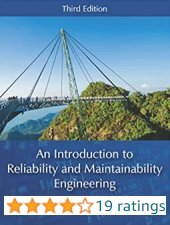An Introduction to Reliability and Maintainability Engineering

Toolkit Home